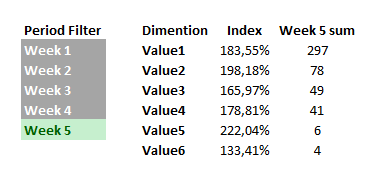# New to Qlik Sense

Discussion board where members can get started with Qlik Sense.

Announcements
BARC’s The BI Survey 19 makes it official. BI users love Qlik. GET REPORTNot applicable

## Calculate a grand, row, column total

Hi,

I'm pretty new to Qlik and this community so i hope i can get advise for solving this calculation problem.

I have a requirement for a KPI  (Index) the formula to calculate it looks like this.

Index =  ( Cell Value X Grand Total) / (Row total X Column Total)Other requirements:

> the output of this calculation will be shown in on one pivot table, with only one row dimension.

> The user will have a week period filter in the report where he can select one or more weeks . The input for the formula has to be the value of the max selected week period (like week 5) and calculate  max 5 weeks back.

I have tried for the grand total the following formula, but the report 'filters' keep messing up the results.

Grand Total: sum({\$<week= {">=\$min(week(day,0) -5 ))<=\$(=max(week(day,0)))"}>} TOTAL facts)

For the Row and Column totals i think an "AGGR" Function is the solution but havent been able to get it working.

Greetings

Jose

3 RepliesMVP

## Re: Calculate a grand, row, column total

May be like this?

Sum(Aggr(

(Sum({\$<week= {">=\$min(week(day,0) -5 ))<=\$(=max(week(day,0)))"}>} facts) *

Sum({\$<week= {">=\$min(week(day,0) -5 ))<=\$(=max(week(day,0)))"}>} TOTAL facts))

/

(Sum({\$<week= {">=\$min(week(day,0) -5 ))<=\$(=max(week(day,0)))"}>} TOTAL <Dimentions> facts) *

Sum({\$<week= {">=\$min(week(day,0) -5 ))<=\$(=max(week(day,0)))"}>} TOTAL <Week> facts))

, Dimentions, Week))Not applicable

## Re: Calculate a grand, row, column total

ah thank you for the fast reply..

I get an output however when i select only one week then it gives me 100% as result.

And i think its only calculating the values from the selected periodes and not going 'back' to a set of max 5 weeks.

I hope this visual helps with the expected output:grMVP

## Re: Calculate a grand, row, column total

How about if you do this

Sum({\$<week= {">=\$min(week(day,0) -5 ))<=\$(=max(week(day,0)))"}>} Aggr(

(Sum({\$<week= {">=\$min(week(day,0) -5 ))<=\$(=max(week(day,0)))"}>} facts) *

Sum({\$<week= {">=\$min(week(day,0) -5 ))<=\$(=max(week(day,0)))"}>} TOTAL facts))

/

(Sum({\$<week= {">=\$min(week(day,0) -5 ))<=\$(=max(week(day,0)))"}>} TOTAL <Dimentions> facts) *

Sum({\$<week= {">=\$min(week(day,0) -5 ))<=\$(=max(week(day,0)))"}>} TOTAL <Week> facts))

, Dimentions, Week))